# Division + reason - math problems

#### Number of problems found: 113

• Beet campaign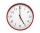The beet's field is being harvested. Beets are transported to 3 plants, 3 trucks. The first car returns to the field 3 times an hour, the second car after 30 minutes, and the third car 5 times an hour. All cars left at 8 in the morning. How many cars do w
• The lift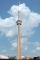The lift can fit four people. How many uphill rides must the lift make to move up 12 passengers?
• Twenty-fiveHow many are three-digit natural numbers divisible by 25?
• By sixFrom the digits 1,2,3,4 we create the long integer number 123412341234. .. .. , which will have 962 digits. Is this number divisible by 6?
• Bdf tablet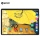Detective Harry Thomson has come across a surprising mystery. As part of the weekend's action, the tablet he looked for dropped by 30%. He also recommended this bargain to his friend. However, it came to the shop on Monday, and the tablet was 30% more exp
• Year 2020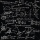The four-digit number divided by 2020 gives a result of 1, **. (Can not be in form 1,*0. ) Write all the options.
• Twos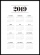Vojta started writing the number of this year 2019202020192020 into the workbook. .. And so he kept going. When he wrote 2020 digits, he no longer enjoyed it. How many twos did he write?
• HexagonDivide a regular hexagon into lines into nine completely identical parts; none of them must be in a mirror image (individual parts can only be rotated arbitrarily).
• Two cars on ring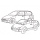There were two cars on the round track (ring) in the adjacent tracks, the first car in the inner track, the second in the outer track. Both cars started at the same time from one starting track. The first toy car drove every four laps simultaneously as th
• Three numbersWe have three different non-zero digits. We will create all 3 digits numbers from them to use all 3 figures in each number. We add all the created numbers, and we get the sum of 1554. What were the numbers?
• Hens and pigs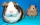Hens and pigs have 46 feet in total. At least how much can heads have?
• Reminder and quotientThere are given the number C = 281, D = 201. Find the highest natural number S so that the C:S, D:S are with the remainder of 1,
• Collection of stampsJano, Rado, and Fero have created a collection of stamps in a ratio of 5: 6: 9. Two of them had 429 stamps together. How many stamps did their shared collection have?
• Four poplarsFour poplars are growing along the way. The distances between them are 35 m, 14 m, and 91 m. At least how many poplars need to be dropped to create the same spacing between the trees? How many meters will it be?
• Bricks pyramidHow many 50cm x 32cm x 30cm brick needed to built a 272m x 272m x 278m pyramid?
• Odd/even numberPick any number. If that number is even, divide it by 2. If it's odd, multiply it by three and add one. Now repeat the process with your new number. If you keep going, you'll eventually end up at one every time. Prove.
• Trees in alleyThere are four trees in the alley between which the distances are 35m, 15m and 95m. Trees must be laid in the spaces so that the distance is the same and the maximum. How many trees will they put in and what will be the distance between them?
• Clock's gears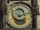In the clock machine, three gears fit together. The largest has 168 teeth, the middle 90 teeth, and the smallest 48 teeth. The middle wheel turns around its axis in 90 seconds. How many times during the day do all the gears meet in the starting position?
• SymbolsIf 2*3 = 60 ; 3*4 = 120 and 4*5 = 200, what is 2*5?
• 7 digit numberIf 3c54d10 is divisible by 330, what is the sum of c and d?

Do you have an exciting math question or word problem that you can't solve? Ask a question or post a math problem, and we can try to solve it.

We will send a solution to your e-mail address. Solved examples are also published here. Please enter the e-mail correctly and check whether you don't have a full mailbox.

Division Problems. Reason - math problems.